# Coulombs Law Charge Q Amount of charge is

• Slides: 9Coulombs LawCharge (Q) Amount of charge is determined by the excess or deficit of electrons Quantity of charge (Q) is measured in coulombs (C ) Ordinary charges are very small and measured in μC (10 -6 C) Charge on electron = -1. 602 x 10 -19 C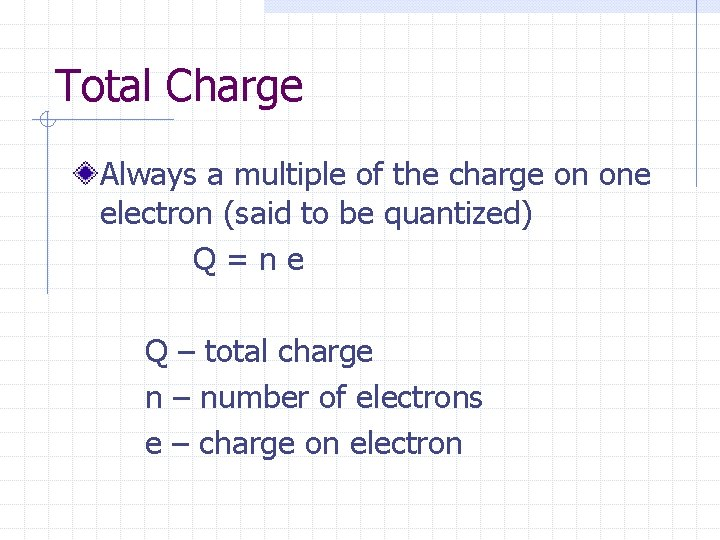Total Charge Always a multiple of the charge on one electron (said to be quantized) Q=ne Q – total charge n – number of electrons e – charge on electron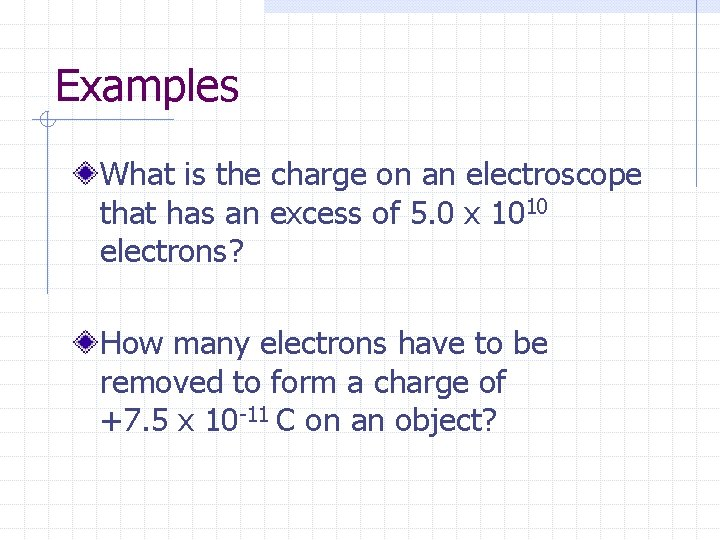Examples What is the charge on an electroscope that has an excess of 5. 0 x 1010 electrons? How many electrons have to be removed to form a charge of +7. 5 x 10 -11 C on an object?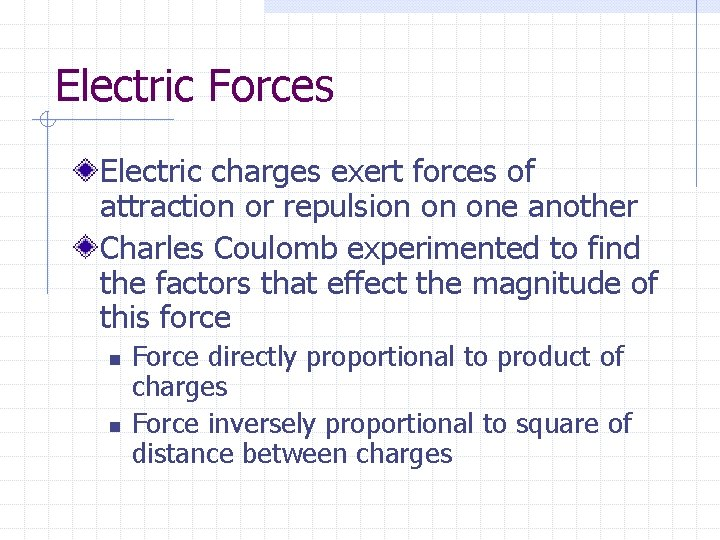Electric Forces Electric charges exert forces of attraction or repulsion on one another Charles Coulomb experimented to find the factors that effect the magnitude of this force n n Force directly proportional to product of charges Force inversely proportional to square of distance between charges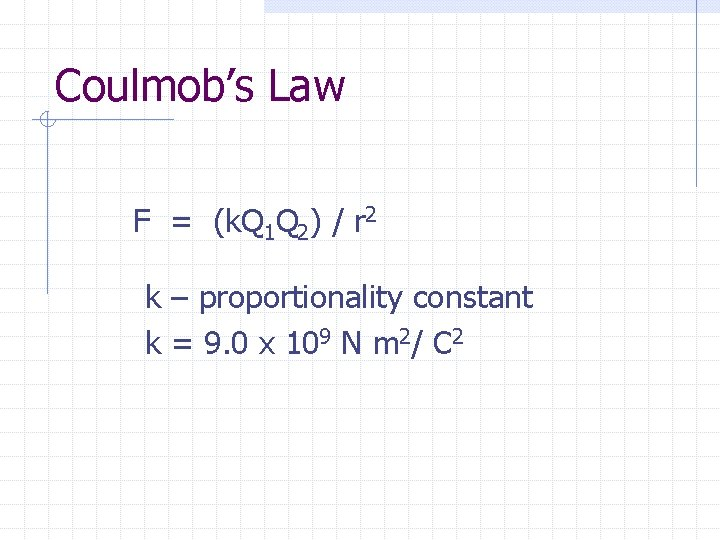Coulmob’s Law F = (k. Q 1 Q 2) / r 2 k – proportionality constant k = 9. 0 x 109 N m 2/ C 2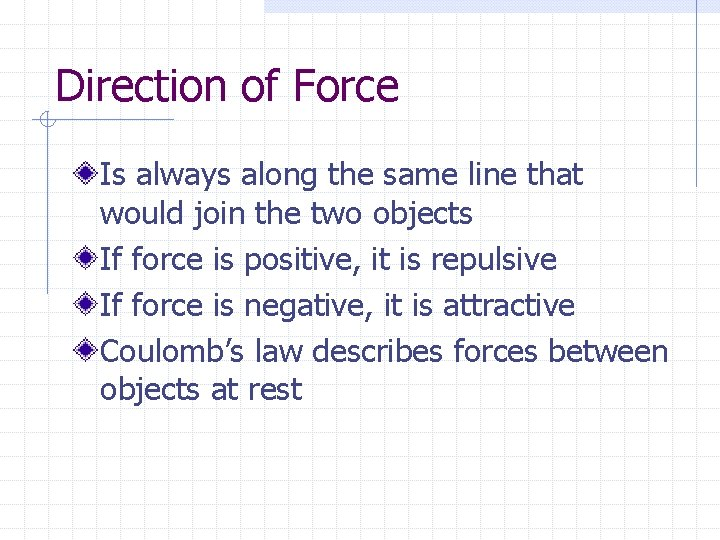Direction of Force Is always along the same line that would join the two objects If force is positive, it is repulsive If force is negative, it is attractive Coulomb’s law describes forces between objects at rest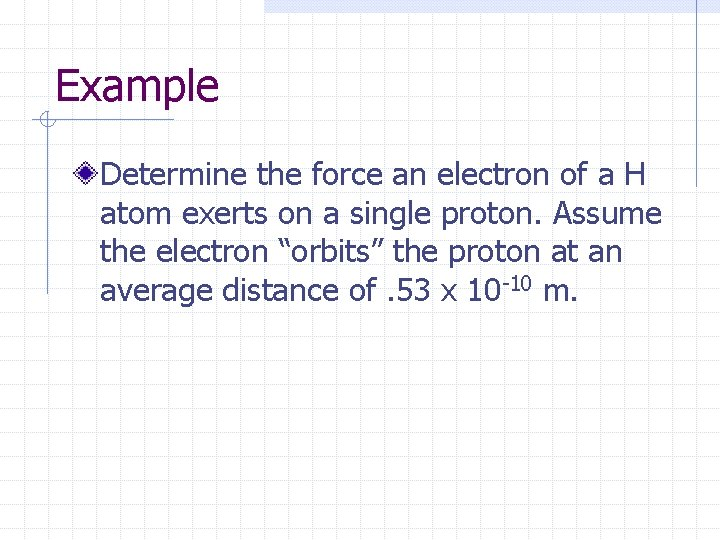Example Determine the force an electron of a H atom exerts on a single proton. Assume the electron “orbits” the proton at an average distance of. 53 x 10 -10 m.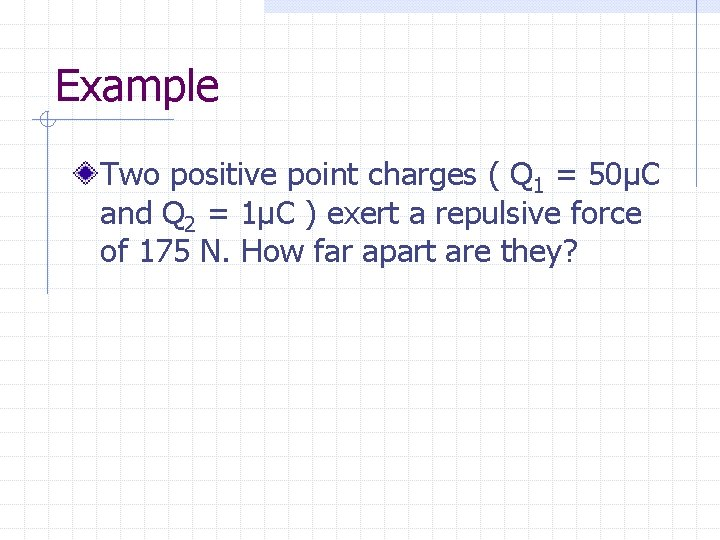Example Two positive point charges ( Q 1 = 50μC and Q 2 = 1μC ) exert a repulsive force of 175 N. How far apart are they?